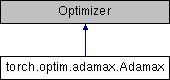Caffe2 - Python API A deep learning, cross platform ML framework## Public Member Functions

def __init__ (self, params, lr=2e-3, betas=(0.9, 0.999), eps=1e-8, weight_decay=0)

def step (self, closure=None)

## Detailed Description

```Implements Adamax algorithm (a variant of Adam based on infinity norm).

It has been proposed in `Adam: A Method for Stochastic Optimization`__.

Arguments:
params (iterable): iterable of parameters to optimize or dicts defining
parameter groups
lr (float, optional): learning rate (default: 2e-3)
betas (Tuple[float, float], optional): coefficients used for computing
running averages of gradient and its square
eps (float, optional): term added to the denominator to improve
numerical stability (default: 1e-8)
weight_decay (float, optional): weight decay (L2 penalty) (default: 0)

__ https://arxiv.org/abs/1412.6980
```

Definition at line 5 of file adamax.py.

## Member Function Documentation

 def torch.optim.adamax.Adamax.step ( self, closure = `None` )
```Performs a single optimization step.

Arguments:
closure (callable, optional): A closure that reevaluates the model
and returns the loss.
```

Definition at line 39 of file adamax.py.

The documentation for this class was generated from the following file: